SBOS557E August   2011  – April 2018

PRODUCTION DATA.

1. Features
2. Applications
3. Description
1.     Device Images
4. Revision History
5. Device Comparison Table
6. Pin Configuration and Functions
7. Specifications
8. Detailed Description
1. 8.1 Overview
2. 8.2 Functional Block Diagram
3. 8.3 Feature Description
4. 8.4 Device Functional Modes
9. Application and Implementation
1. 9.1 Application Information
2. 9.2 Typical Application
10. 10Power Supply Recommendations
11. 11Layout
12. 12Device and Documentation Support
1. 12.1 Device Support
1. 12.1.1 Third-Party Products Disclaimer
2. 12.1.2 Development Support
2. 12.2 Documentation Support
5. 12.5 Community Resources
7. 12.7 Electrostatic Discharge Caution
8. 12.8 Glossary
13. 13Mechanical, Packaging, and Orderable Information

• D|8
• DSG|8
• DGK|8
• DCU|8
• D|8
• DSG|8
• DGK|8
• DCU|8

### 7.8 Typical Characteristics

VS = ±18 V, VCM = VS / 2, RLOAD = 10 kΩ connected to VS / 2, and CL = 100 pF, (unless otherwise noted)

### Table 1. Characteristic Performance Measurements

DESCRIPTION FIGURE
Offset Voltage Production Distribution Figure 1
Offset Voltage Drift Distribution Figure 2
Offset Voltage vs Temperature Figure 3
Offset Voltage vs Common-Mode Voltage Figure 4
Offset Voltage vs Common-Mode Voltage (Upper Stage) Figure 5
Offset Voltage vs Power Supply Figure 6
IB and IOS vs Common-Mode Voltage Figure 7
Input Bias Current vs Temperature Figure 8
Output Voltage Swing vs Output Current (Maximum Supply) Figure 9
CMRR and PSRR vs Frequency (Referred-to-Input) Figure 10
CMRR vs Temperature Figure 11
PSRR vs Temperature Figure 12
0.1-Hz to 10-Hz Noise Figure 13
Input Voltage Noise Spectral Density vs Frequency Figure 14
THD+N Ratio vs Frequency Figure 15
THD+N vs Output Amplitude Figure 16
Quiescent Current vs Temperature Figure 17
Quiescent Current vs Supply Voltage Figure 18
Open-Loop Gain and Phase vs Frequency Figure 19
Closed-Loop Gain vs Frequency Figure 20
Open-Loop Gain vs Temperature Figure 21
Open-Loop Output Impedance vs Frequency Figure 22
Small-Signal Overshoot vs Capacitive Load (100-mV Output Step) Figure 23, Figure 24
No Phase Reversal Figure 25
Small-Signal Step Response (100 mV) Figure 28, Figure 29
Large-Signal Step Response Figure 30, Figure 31
Large-Signal Settling Time (10-V Positive Step) Figure 32
Large-Signal Settling Time (10-V Negative Step) Figure 33
Short-Circuit Current vs Temperature Figure 34
Maximum Output Voltage vs Frequency Figure 35
EMIRR IN+ vs Frequency Figure 36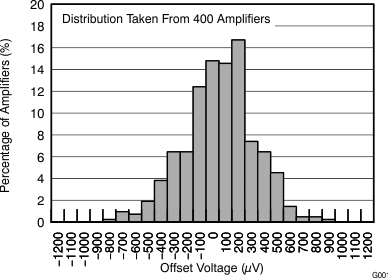Figure 1. Offset Voltage Production Distribution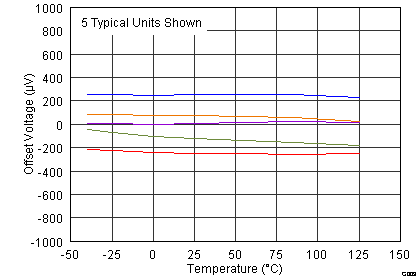Figure 3. Offset Voltage vs Temperature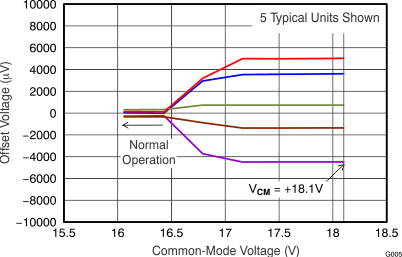Figure 5. Offset Voltage vs Common-Mode Voltage
(Upper Stage)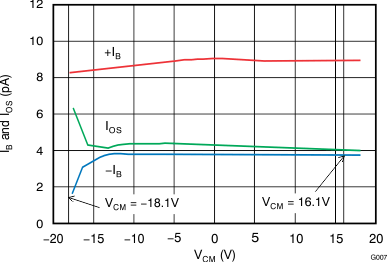Figure 7. IB and IOS vs Common-Mode VoltageFigure 9. Output Voltage Swing vs Output Current (Maximum Supply)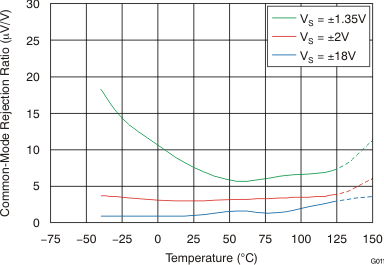Figure 11. CMRR vs Temperature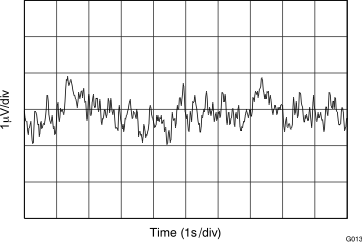Figure 13. 0.1-Hz to 10-Hz Noise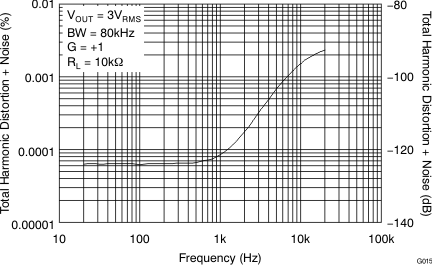Figure 15. THD+N Ratio vs Frequency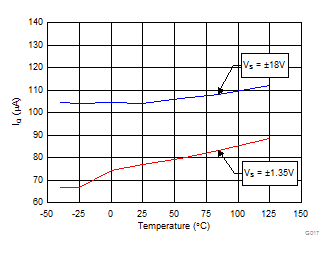Figure 17. Quiescent Current vs Temperature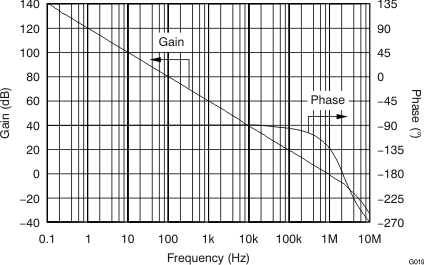Figure 19. Open-Loop Gain and Phase vs Frequency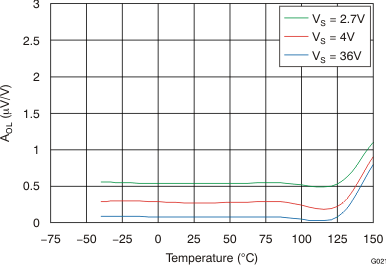Figure 21. Open-Loop Gain vs Temperature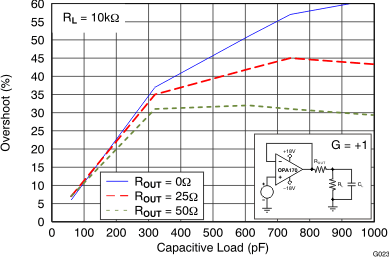100-mV output step
Figure 23. Small-Signal Overshoot vs Capacitive Load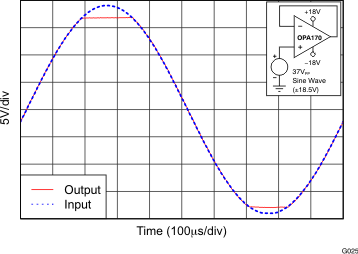Figure 25. No Phase Reversal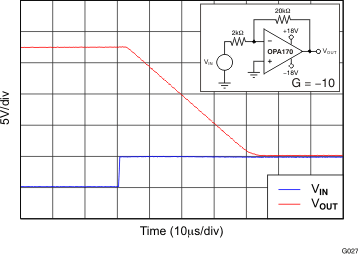Figure 27. Negative Overload Recovery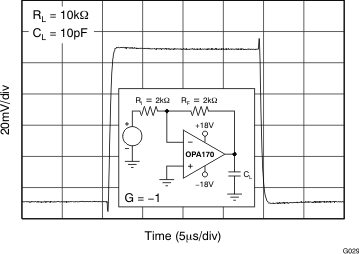Figure 29. Small-Signal Step Response (100 mV)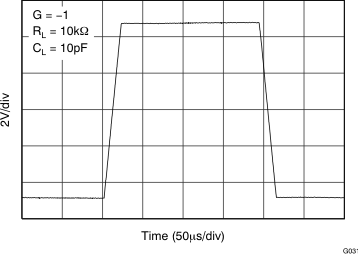Figure 31. Large-Signal Step Response10-V negative step
Figure 33. Large-Signal Settling Time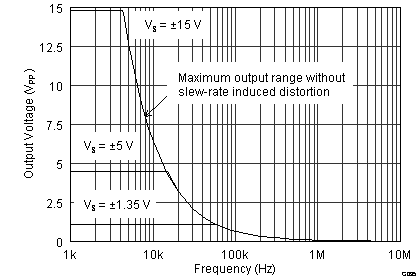Figure 35. Maximum Output Voltage vs FrequencyFigure 2. Offset Voltage Drift DistributionFigure 4. Offset Voltage vs Common-Mode Voltage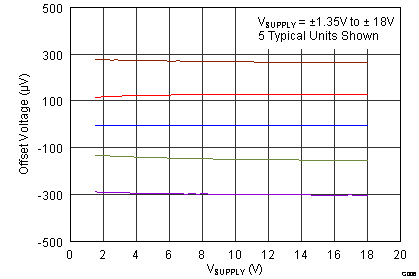Figure 6. Offset Voltage vs Power Supply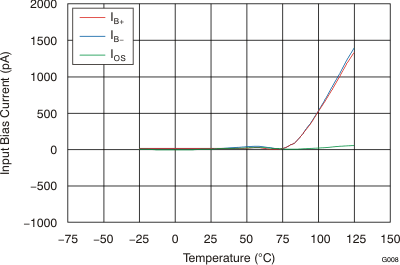Figure 8. Input Bias Current vs Temperature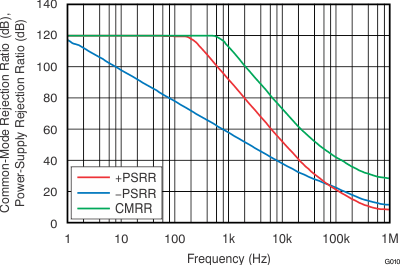Figure 10. CMRR and PSRR vs Frequency
(Referred-to Input)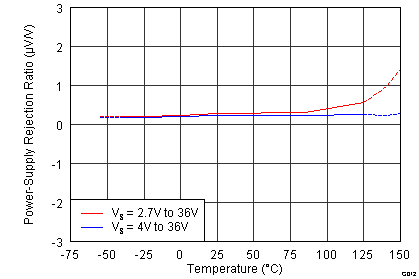Figure 12. PSRR vs Temperature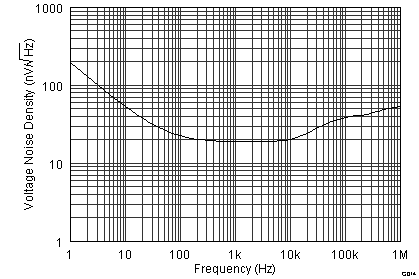Figure 14. Input Voltage Noise Spectral Density vs Frequency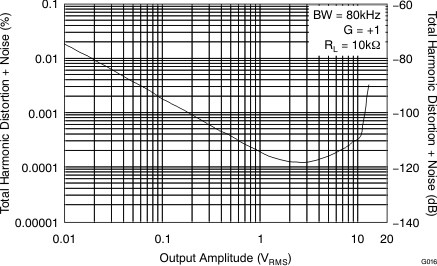Figure 16. THD+N vs Output Amplitude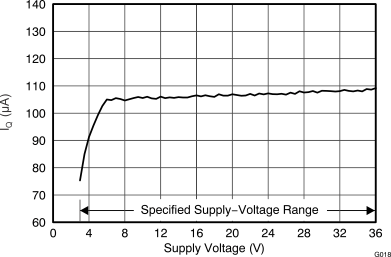Figure 18. Quiescent Current vs Supply Voltage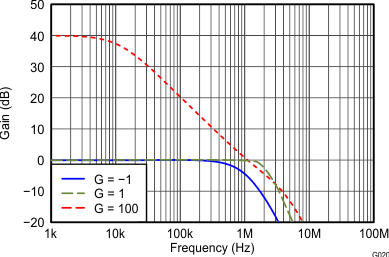Figure 20. Closed-Loop Gain vs Frequency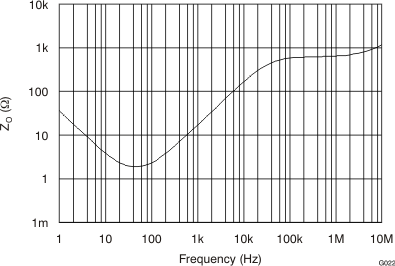Figure 22. Open-Loop Output Impedance vs Frequency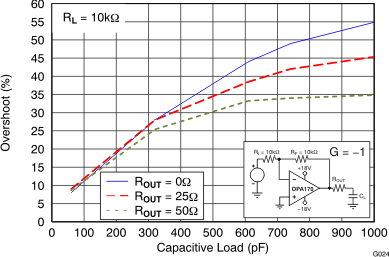100-mV output step
Figure 24. Small-Signal Overshoot vs Capacitive Load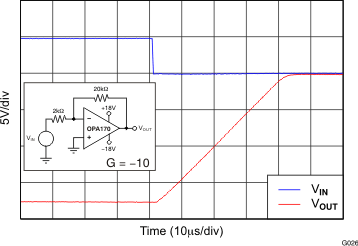Figure 26. Positive Overload Recovery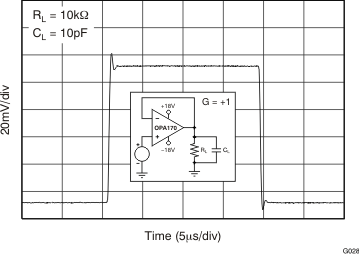Figure 28. Small-Signal Step Response (100 mV)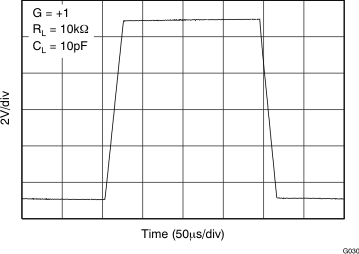Figure 30. Large-Signal Step Response10-V positive step
Figure 32. Large-Signal Settling Time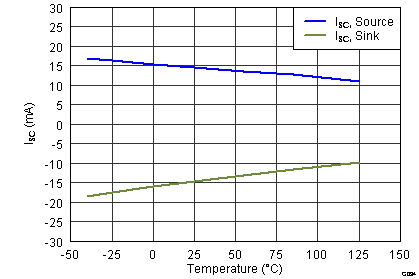Figure 34. Short-Circuit Current vs Temperature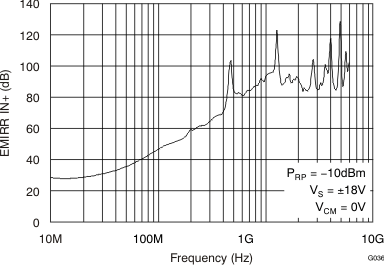Figure 36. EMIRR IN+ vs Frequency